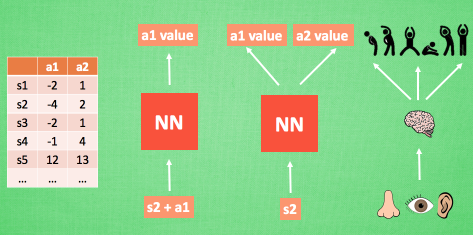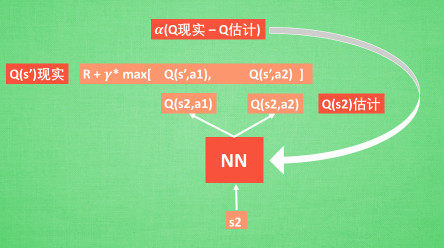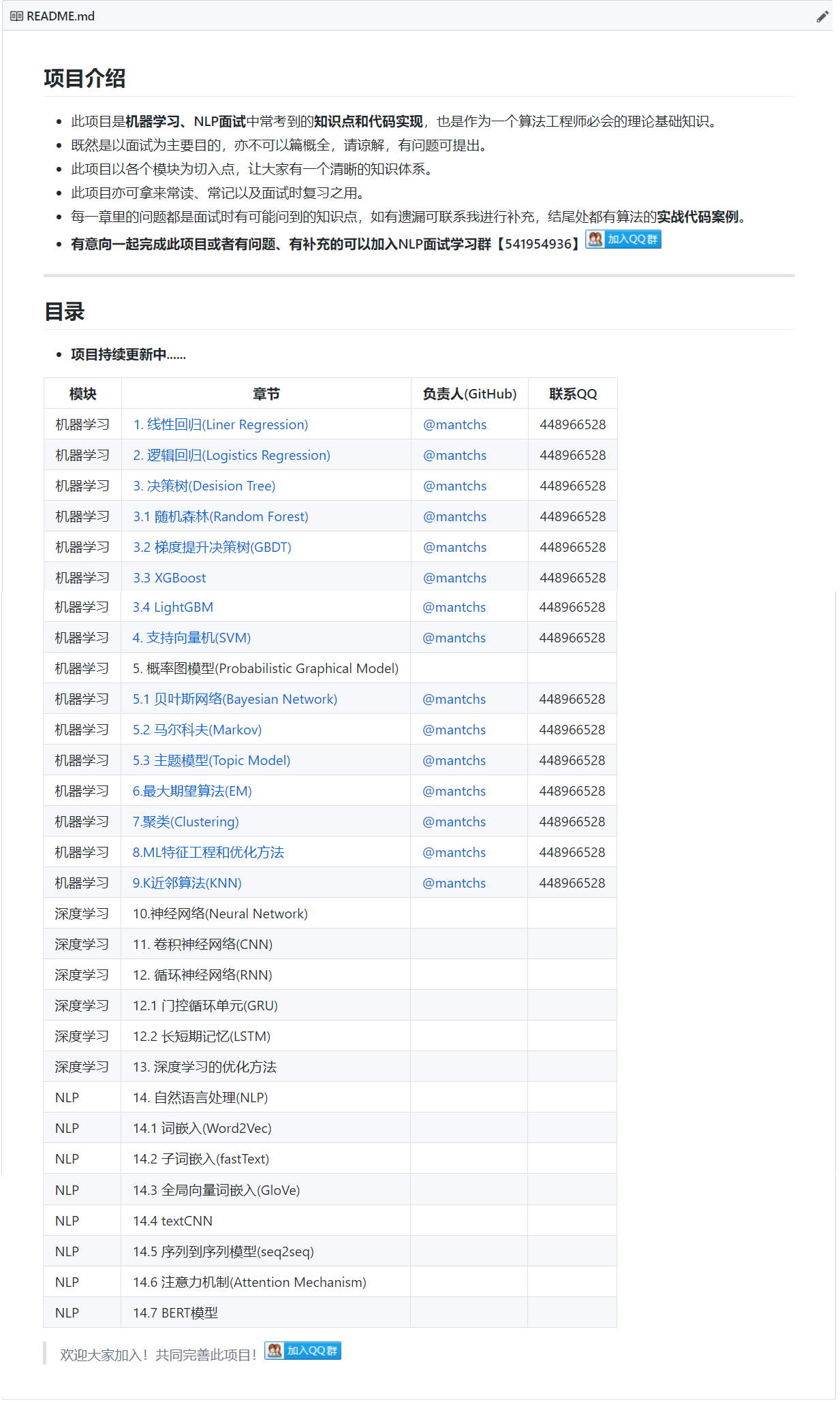# 【深度学习】强化学习

## 1. 什么是强化学习

1. 基本是以一种闭环的形式；
2. 不会直接指示选择哪种行动（actions）；
3. 一系列的actions和奖励信号（reward signals）都会影响之后较长的时间。1. 做的好（reward）即正反馈，
2. 做得不好（punishment惩罚）即负反馈。

Agent可能做得好，也可能做的不好，环境始终都会给它反馈，agent会尽量去做对自身有利的决策，通过反反复复这样的一个循环，agent会越来越做的好，就像孩子在成长过程中会逐渐明辨是非，这就是强化学习。

## 2. 强化学习模型• 当前状态 St 只由上一个状态 St-1 和行为所决定，而和前序的更多的状态是没有关系的。

### 2.1 打折的未来奖励

$$R=r_1+r_2+r_3+…+r_n$$

t 时刻(当下)的未来奖励，只考虑后面的奖励，前面的改变不了：

$$R_t=r_t+r_{t+1}+r_{t+2}+…+r_n$$

$$R_t=r_1+\gamma_{}r_{t+1}+\gamma^2r_{t+2}+…+\gamma^{n-1}r_n$$

Rt 可以用 Rt+1 来表示，写成递推式：

$$R_t=r_t+\gamma(r_{t+1}+\gamma(r_{t+2}+…))=r_t+\gamma_{}R_{t+1}$$

### 2.2 Q-Learning算法

Q(s, a)函数(Quality)，质量函数用来表示智能体在s状态下采用a动作并在之后采取最优动作条件下的打折的未来奖励(先不管未来的动作如何选择)：

$$Q(s_t,a_t)=maxR_{t+1}$$

$$\pi(s)=argmax_aQ(s,a)$$

$$Q(s_t,a_t)=r+\gamma_{}max_aQ(s_{t+1},a_{t+1})$$

Q-learning的核心思想是：我们能够通过贝尔曼公式迭代地近似Q-函数。

### 2.3 Deep Q Learning(DQN)

Deep Q Learning(DQN)是一种融合了神经网络和的Q-Learning方法。

#### 2.3.1 神经网络的作用#### 2.3.2 神经网络计算Q值## 3. 强化学习和监督学习、无监督学习的区别

1. 监督式学习就好比你在学习的时候，有一个导师在旁边指点，他知道怎么是对的怎么是错的。

强化学习会在没有任何标签的情况下，通过先尝试做出一些行为得到一个结果，通过这个结果是对还是错的反馈，调整之前的行为，就这样不断的调整，算法能够学习到在什么样的情况下选择什么样的行为可以得到最好的结果。

2. 监督式学习出的是之间的关系，可以告诉算法什么样的输入对应着什么样的输出。监督学习做了比较坏的选择会立刻反馈给算法。

强化学习出的是给机器的反馈 reward function，即用来判断这个行为是好是坏。 另外强化学习的结果反馈有延时，有时候可能需要走了很多步以后才知道以前的某一步的选择是好还是坏。

3. 监督学习的输入是独立同分布的。

强化学习面对的输入总是在变化，每当算法做出一个行为，它影响下一次决策的输入。

4. 监督学习算法不考虑这种平衡，就只是 exploitative。

强化学习，一个 agent 可以在探索和开发（exploration and exploitation）之间做权衡，并且选择一个最大的回报。

5. 非监督式不是学习输入到输出的映射，而是模式(自动映射)。

对强化学习来说，它通过对没有概念标记、但与一个延迟奖赏或效用（可视为延迟的概念标记）相关联的训练例进行学习，以获得某种从状态到行动的映射。

## 4. 什么是多任务学习## 5. 参考文献